Courses

# Long Answer Type Questions(Part- 2)- Statistics Class 10 Notes | EduRev

## Class 10 : Long Answer Type Questions(Part- 2)- Statistics Class 10 Notes | EduRev

The document Long Answer Type Questions(Part- 2)- Statistics Class 10 Notes | EduRev is a part of the Class 10 Course Mathematics (Maths) Class 10.
All you need of Class 10 at this link: Class 10

Q11. During the medical checkup of 35 students of a class, their weights were recorded as follows:

 Weight (in kg) Number of students 38-40 3 40-42 2 42-44 4 44-46 5 46-48 14 48-50 4 50-52 3

Draw a ‘less than type’ and ‘more than type’ ogive from the given data. Hence, obtain the median weight from the graph.

Sol.

 Weight (in kg) No. of students Less than cumulative frequency More than cumulative frequency 38-40 3 Less than 40 = 3 38 and more than 38 = 35 40-42 2 Less than 42 = 5 40 and more than 40 = 32 42-44 4 Less than 44 = 9 42 and more than 42 = 30 44-46 5 Less than 46 = 14 44 and more than 44 = 26 46-48 14 Less than 48 = 28 46 and more than 46 = 21 48-50 4 Less than 50 = 32 48 and more than 48 = 7 50-52 3 Less than 52 = 35 50 and more than 50 = 3 Total ∑ fi = 35 ⇒ n = 35

∴ For less than type ogive, we plot the points (40, 3), (42, 5), (44, 9), (46, 14), (48, 28), (50, 32) and (52, 35).
∴ For more than type ogive, we plot the points (38, 35), (40, 32), (42, 30), (44, 26), (46, 21), (48, 7) and (50, 3) on the same graph.
From the graph, we obtain the median = 46.5 kg.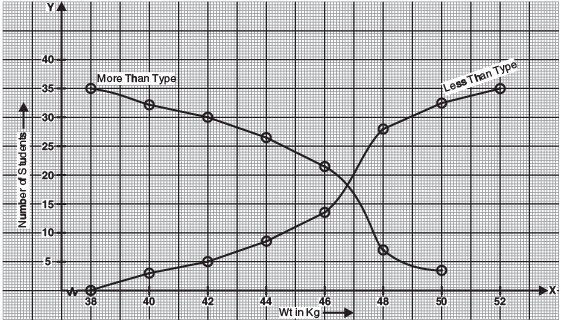Q12. The following table gives daily income of 50 workers of a factory:

 Daily income (in Rs) 100-120 120-140 140-160 160-180 180-200 Number of workers 12 14 8 6 10

Find the mean, mode and median of the above data.

Sol. Let assumed mean a = 150. Here, h = 20

 Daily income (in Rs) No. of workers (fi) xi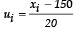fi ui cf 100-120 12 110 -2 -24 12 + 0 = 12 120-140 14 130 -1 -14 12 + 14 = 26 140-160 8 150 0 0 26 + 8 = 34 160-180 6 170 1 6 34 + 6 = 40 180-200 10 190 2 20 40 + 10 = 50 Total ∑ fi = 50 ∑ fiui  =-12

(i) Mean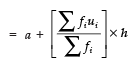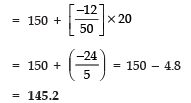Thus, mean income is Rs 145.2

(ii) For finding the mode,
We have the greatest frequency = 14 which lies in the class 120−140
∴ Modal class = 120−140
Therefore, l = 120
f1 = 14
f0 = 12
f2 = 8
and h = 20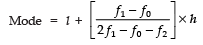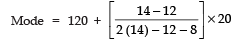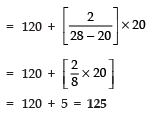(iii) For finding median,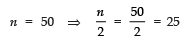And 25 lies in the class 120−140

Median class is 120−140

Since n/2 = 25, cf = 12, f = 14 and h = 20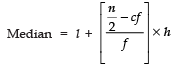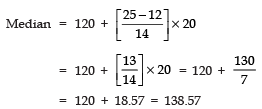Median income = Rs138.57

Q13. Find the mode, median and mean for the following data:

 Marks obtained 25-35 35-45 45-55 55-65 65-75 75-85 Number of students 7 31 33 17 11 1

Sol. Let the assumed mean a = 60. Here h = 10, we have:

 Marksobtained Class marksxi fi cf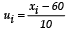fi ui 25-35 30 7 7 -3 -21 35-45 40 31 38 -2 -62 45-55 50 33 71 -1 -33 55-65 60 17 88 0 0 65-75 70 11 99 1 11 75-85 80 1 100 2 2 Total ∑ f = 100 ⇒ n = 100 ∑ fiui =-103

(i)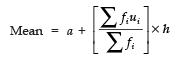⇒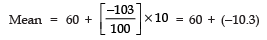(ii) Median

Here,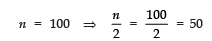∴ Median class is 45−55.

l = 45
cf = 38
f = 33 and h = 10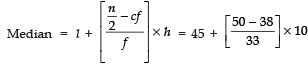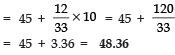(iii) Mode: Greatest frequency is 33 which corresponds to the class 45−55.

l = 45,  h = 10
f1 = 33,  f2 = 17
f0 = 31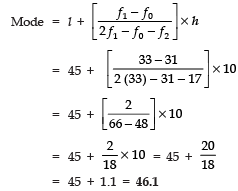Q14. A survey regarding the heights (in cm) of 50 girls of Class X of a school was conducted and the following data was obtained:

 Height (in cm) 120-130 130-140 140-150 150-160 160-170 Total Number of girls 2 8 12 20 8 50

Find the mean, median and mode of the above data.

Sol. We have:

 Height (in cm) f cf xi fixi 120-130 2 2 + 0 = 2 125 250 130-140 8 2 + 8 = 10 135 1080 140-150 12 10 + 12 = 22 145 1740 150-160 20 22 + 20 = 42 155 3100 160-170 8 42 + 8 = 50 165 1320 Total 50 7490

(i)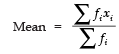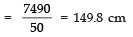(ii) ∵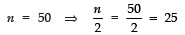∴ Median class is 150−160.
∴ We have,
l = 150
f = 20
cf = 2
2h = 10

∴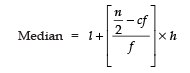⇒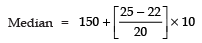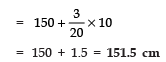(iii) ∵ Greatest frequency = 20
∴ Modal class = 150−160

So, we have

l = 150, f0 = 12, f1 = 20
f2 = 8 and h = 10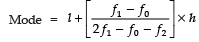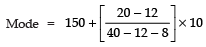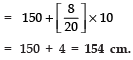Q15. Find the mean, mode and median of the following data:

 Classes Frequency 0-10 5 10-20 10 20-30 18 30-40 30 40-50 20 50-60 12 60-70 5

Sol.

(i) Mean:

Let the assumed mean ‘a’ = 35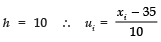Now we have the following data:

 Class Class mark xi fi Cf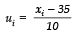fiui 0-10 5 5 5 + 0 = 5 -3 (-3) x 5 = -15 10-20 15 10 5 + 10 = 15 -2 (-2) x 10 = -20 20-30 25 18 15 + 18 = 33 -1 (-1) x 18 = -18 30-40 35 30 33 + 30 = 63 0 0 x 30 = 0 40-50 45 20 63 + 20 = 93 1 1 x 20 = 20 50-60 55 12 83 + 12 = 95 2 2 x 12 = 24 60-70 65 5 95 + 5 = 100 3 3 x 5 = 15

Here ∑fi = 100 and ∑fiui = 6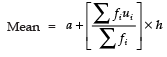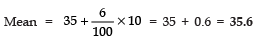(ii) Mode:

Here, the maximum frequency is 30.
∴ Modal class is 30−40.
So, we have

l = 30, h = 10
f1 = 30,
f0 = 18
f2 = 20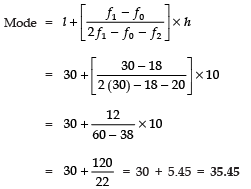(iii) Median: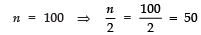∴ Median class = 30−40
So, we have:

l = 30, h = 10, cf = 33, f = 30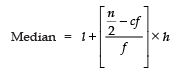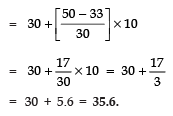Q16. Find the mean, mode and median for the following data:

 Classes Frequency 0-10 3 10-20 8 20-30 10 30-40 15 40-50 7 50-60 4 60-70 3

Sol. Let the assumed mean = 35;  h = 10

 Classes xi fi cf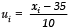fiui 0-10 5 3 3 + 0 = 3 -3 (-3) x 3 = -9 10-20 15 8 3 + 8 = 11 -2 (-2) x 8 = -16 20-30 25 10 11 + 10 = 21 -1 (-1) x 10 = -10 30-40 35 15 21 + 15 = 36 0 0 x 15 = 0 40-50 45 7 36 + 7 = 43 1 1 x 7= 7 50-60 55 4 43 + 4 = 47 2 2 x 4= 8 60-70 65 3 47 + 3 = 50 3 3 x 3= 9 Total ∑fi = 50 ∑fiui= -11

Now,

(i)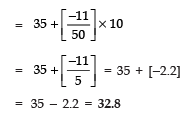(ii) To find mode
Here, highest frequency is 15.
∴ Modal class is 30−40.

Here,

l = 30, f1 = 15, f0 = 10
f2 = 7 and h = 10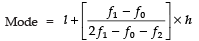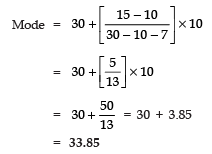(iii) ∵So, the median class is 30−40.

∴ We have l = 30, cf = 21, f = 15 and h = 10

We have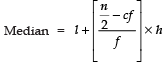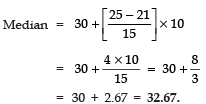Q18. Find the mean, mode and median of the following data:

 Class Frequency 0-10 3 10-20 4 20-30 7 30-40 15 40-50 10 50-60 7 60-70 4

Sol. Let the assumed mean ‘a’ = 35

Here, h =10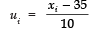Classes xi fi cf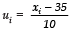fiui 0-10 5 3 3 + 0 = 3 -3 (-3) x 3 = -9 10-20 15 4 4 + 3 = 7 -2 (-2) x 4 = -8 20-30 25 7 7 + 7 = 14 -1 (-1) x 7 = -7 30-40 35 15 15 + 14 = 29 0 0 x 15 = 0 40-50 45 10 10 + 29 = 39 1 1 x 10 = 10 50-60 55 7 7 + 39 = 46 2 2 x 7 = 14 60-70 65 4 4 + 46 = 50 3 3 x 4 = 12 Total ∑fi = 50 ∑uifi = 12

(i)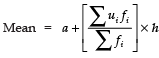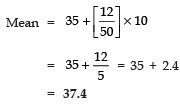(ii) To find mode
Here, greatest frequency = 15
∴ Modal class = 30 − 40
l = 30, f1 = 15, f0 = 7, f2 = 10 and h = 10

So,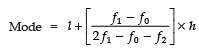⇒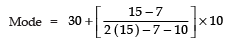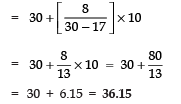(iii) To find median

Here,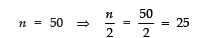∴ Median class is 30−40.
Such that l = 30, cf = 14, f = 15 and h = 10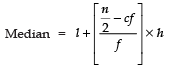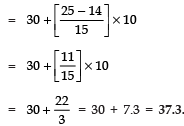Offer running on EduRev: Apply code STAYHOME200 to get INR 200 off on our premium plan EduRev Infinity!

## Mathematics (Maths) Class 10

50 videos|346 docs|103 tests

,

,

,

,

,

,

,

,

,

,

,

,

,

,

,

,

,

,

,

,

,

;